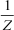HR
【判断题】单词“refrain”既可以作名词表示“副歌”,也可以作动词表示“克制”。
A.

B.手机使用分享复制链接新浪微博分享QQ微信扫一扫反馈( )不符合实验室仪器设备管理要求。
A.

B.

C.

D.

A.

B.

C.

D.

A.

B.

C.

D.

A.

B.

C.

D.

A.

B.

C.

D.

A.

B.

C.

D.

A.

B.

C.

D.

A.
15
B.
14
C.
13
D.
12

A.

B.

C.

D.

A.
20g/L碳酸氢钠
B.
20g/L硼酸
C.
20g/L硼砂D、20g/L碳酸钠

A.

B.

C.

D.

( )应当制定粮食质量安全事故处置方案。
A.

B.

C.

D.

A.

B.

C.

D.

A.

B.

C.

D.

[H+]=2.0×10-5mol/L，按对数的有效数字运算规则， 其 pH 值应为( )。
A.
4.699
B.
4.7
C.
4.70
D.
4.700

A.
B.

C.

D.

A.

B.

C.

D.

A.

B.

C.

D.

A.
95%-105%
B.
90%-110%
C.
80%-110%
D.
60%-120%

A.

B.

C.

D.

A.

B.

C.

D.

A.

B.

C.

D.

《食品安全国家标准 植物油》 GB 2716-2018 对二级食用植物油中溶剂残留量的规定是( )。
A.
≤10 mg/kg
B.
≤20 mg/kg
C.
≤60mg/kg
D.
≤100 mg/kg

A.

B.

C.

D.

A.

B.

C.

D.

4 号码在 9 处，该油脂样品的相对密度为()。
A.
0.9279
B.
9.279
C.
92.79
D.
0.9729

A.
50
B.
40
C.
30
D.
20
《中华人民共和国食品安全法》规定， 食品检验由( ) 指定的检验人独立进行。
A.

B.

C.

D.

A.
90%
B.
80%
C.
70%
D.
60%

A.

B.

C.

D.

A.

B.

C.

D.

A.
0.1:1
B.
(0.2~0.5):1
C.
1:1
D.
2:1

A.

B.

C.

D.

A.

B.

C.

D.

A.

B.

C.

D.

A.

B.

C.

D.

A.

B.

C.

D.

A.

B.

C.

D.

A.
B.

C.

D.

A.

B.

C.

D.

A.
Q 检验法
B.
F 检验法
C.
F 检验加 t 检验法
D.
t 检验法

A.

B.

C.

D.

A.
9mol/L 硫酸
B.
6mol/L 盐酸
C.

D.

A.

B.

C.

D.

A.
20目
B.
40 目
C.
80 目
D.
100 目

A.
1/10点
B.
2/10 点
C.
3/10 点
D.

A.

B.

C.

D.

A.
30%以上
B.
10%~25%
C.
1%~10%
D.

A.
1.5g
B.
2g
C.
3g
D.
5g

A.
41
B.
51
C.
61
D.
71

A.

B.

C.

D.

A.

B.

C.

D.

A.

B.

C.

D.

A.

B.

C.

D.

A.

B.

C.

D.

A.

B.

C.

D.

A.

B.

C.

D.

A.
0.1g
B.
0.2g
C.
0.3g
D.
0.4g

A.
2.70
B.
2.71
C.
1.57
D.
1.58

A.

B.

C.

D.

A.

B.

C.

D.

A.
400~500F.U.之间
B.
480~520F.U.之间
C.
500~600F.U.之间
D.
600~1000F.U.之间
EDTA 的化学名称为(有较高的稳定性。
A.

B.

C.
N-1-萘基乙二胺 )，它能与大多数金属离子形成环状的螯合物， 具
D.

A.

B.

C.

D.

A.

B.

C.

D.
Z·M(B) =M(B)

A.
1
B.
1.5
C.
2
D.
3

A.

B.

C.

D.

A.
160
B.
554
C.
900
D.
1600

A.

B.

C.

D.

A.
1g
B.
2g
C.
3g
D.
4g

A.

B.

C.

D.

A.
20mm
B.
30mm
C.
40mm
D.
20mm

A.

B.

C.

D.

A.
65%以上
B.
65%-70%
C.
70%-75%
D.
65%-75%

A.

B.

C.

D.

A.

B.

C.

D.

A.

B.

C.

D.

A.

B.
10℃-20℃
C.
10℃-25℃
D.
15℃-25℃

A.
1:1
B.
3:1
C.
3:4
D.
1:3

A.

B.

A.

B.

C.

D.

A.
3.0%
B.
4.0%
C.
30 μmol/g
D.
35 μmol/g

A.

B.

C.

D.

A.
B.

C.
PBS溶液(pH=7.0)
D.
PBS 溶液( pH=6.5 )

A.

B.

C.

D.

A.

B.

C.

D.

A.

B.

C.

D.

A.
0.07
B.
0.06
C.
0.068
D.
0.1

A.

B.

C.

D.

A.

B.

C.

D.

A.

B.

C.

D.

A.

B.

C.

D.

A.

B.

C.

D.

A.

B.

C.

D.

HPLC法分离糖类时，常采用( )作为固定相。
A.
C18 键合固定相
B.

C.

D.

A.

B.

C.

D.

A.

B.

C.

D.

A.

B.

C.

D.121%